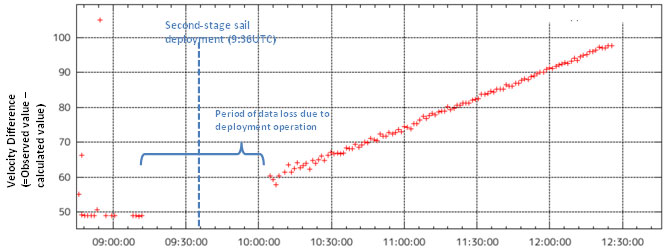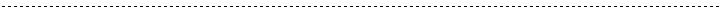## Small Solar Power Sail Demonstrator 'IKAROS' Confirmation of Photon Acceleration

July 9, 2010 (JST)

Japan Aerospace Exploration Agency (JAXA)

The Japan Aerospace Exploration Agency (JAXA) would like to announce that we have confirmed the successful acceleration of the Small Solar Power Sail Demonstrator "IKAROS" by photon (*1) in the course of determining its precise orbit after its sail deployment. The IKAROS was launched by JAXA on May 21, 2010 (Japan Standard time, and all the following time and dates are JST unless noted otherwise,) and has been under operation since then.
The thrust by solar light pressure is 1.12 mili-Newton (*2,) which is the expected value.
With this confirmation, the IKAROS was proved to generate the biggest acceleration through photon during interplanetary flight in history.

*1 Photon acceleration is to accelerate an object by pressure of solar photon (solar light pressure.)
*2 Newton (N) is a unit that shows the amount of net force required to accelerate a mass of one kilogram at a rate of one meter per second per second. In other words, it indicates the thrust power of the demonstrator. 1.12 mN is equivalent to about 0.114 g of gravity loaded onto an object on the Earth.

Figure 1 Doppler Measurement at the Second-stage Sail DeploymentTime on June 6 (Universal Time)

The above Figure 1 shows the difference between calculated value (without photon acceleration) and observed value (result of Doppler measurement) of IKAROS's line-of-sight velocity (*3) on June 9, when the second-stage sail deployment was carried out. The calculated value does not incorporate photon acceleration, thus, the graph shows a flat line if there is no photon acceleration. With photon acceleration, the line inclines. For about one hour around 9:36 (Universal Time,) when the second-stage deployment was in operation, data was lost; however, you can clearly see that the graph line starts inclining after the second-stage deployment compared to the flat line prior to the event.

(*3) Line-of-sight velocity is a velocity with direction components connecting a ground station and the IKAROS among flight velocities relative to the ground.

Figure 2 Estimated Parameters Based on IKAROS Orbit Determination ResultSail's effective cross section area ratio = the estimated sail area divided by the area at the time of manufacturing Sail specular coefficient = amount of secularly reflected photons divided by total incident photons

Figure 2 compares the IKAROS's orbit determination result and sail's optical parameters at the time of it's manufacture (namely sail's effective cross section area ratio and sail's specular coefficient) since June 9.
The estimated thrust by solar light pressure due to the orbit determination this time is 1.12 mN (mili-Newton,) and the sky blue curve line in Figure 2 shows the combination of the sail's optical parameters to realize the estimated thrust.
The area in the square in Figure 2 shows the estimated parameter range based on the design values at the time of sail manufacturing. (The sail's shape is deformed by light pressure in orbit, thus the estimated value is dispersed from the design value as you can see in Figure 2.)
As Figure 2 shows, the IKAROS exhibits its photon acceleration capability as designed.